# Lecture 24: Second-order optimization¶

Overview:

In :
%matplotlib inline

import numpy as np
import matplotlib
import matplotlib.pyplot as plt
import sklearn.linear_model as linear_model

np.random.seed(1337)
from timeit import timeit

from plotters import error_plot, convergence_plot, kwargs, setup_layout

setup_layout()


## Newton iteration¶

Below is a naive implementation of Newton's iteration that we analyzed in the last lecture.

In :
def newton_method(init, steps, grad, hessian, num_to_keep=None):
"""Newton iteration with full Hessian inverse.

Parameters
----------
initial : array
starting point
steps : list of floats
step size schedule for the algorithm
mapping arrays to arrays of same shape
hessian : function
mapping 1d arrays to 2d arrays
num_to_keep : integer, optional
number of points to keep

Returns
-------
List of points computed by Newton iteration. Length of the
list is determined by num_to_keep.
"""
xs = [init]
for step in steps:
Hinv = np.linalg.pinv(hessian(xs[-1]))
if num_to_keep:
xs = xs[-num_to_keep:]
return xs


As a sanity check, Newton's method should solve a quadratic in one step. The same is true for least squares.

In :
def least_squares(A, b, x):
"""Least squares objective."""
return (0.5/len(A)) * np.linalg.norm(A.dot(x)-b)**2

"""Gradient of least squares objective at x."""
return A.T.dot(A.dot(x)-b)/len(A)

def least_squares_hessian(A, x):
"""Hessian of least squares objective."""
return A.T.dot(A)/len(A)

In :
m, n = 1000, 100
A = np.random.normal(0, 1, (m, n))
x_opt = np.random.normal(0, 1, n)
noise = np.random.normal(0, 0.1, m)
b = A.dot(x_opt)
objective = lambda x: least_squares(A, b, x)
hessian = lambda x: least_squares_hessian(A, x)
x0 = np.random.normal(0, 1, n)

In :
xs = newton_method(x0, , gradient, hessian)
errors = [objective(x) for x in xs]

In :
error_plot(errors)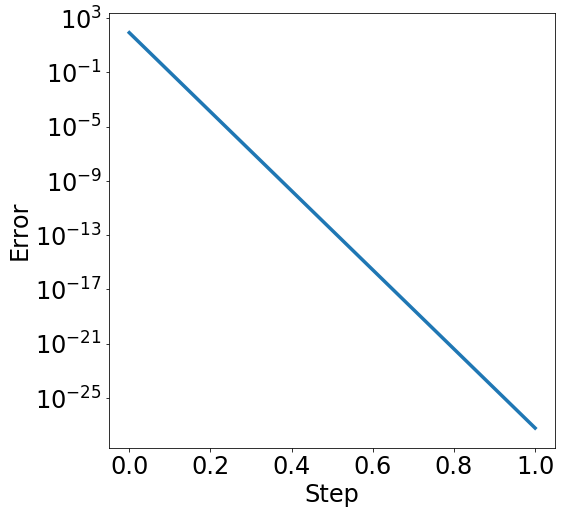## Logistic regression¶

Let's move on to a less trivial example that we'll stick to for the rest of the lecture: logistic regression. A logistic model makes predictions based on a sigmoid function applied to a linear function of the data.

In :
def sigmoid(x):
"""Sigmoid function."""
return 1./(1.+np.exp(-x))

In :
xs = np.linspace(-5, 5, 100)
plt.plot(xs, sigmoid(xs), **kwargs);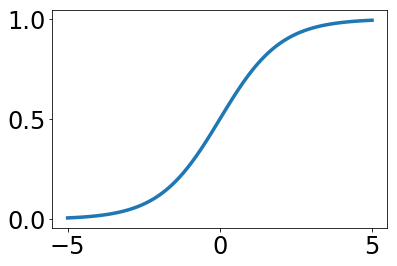In :
def logistic_model(weights, data):
"""Logistic model."""
return sigmoid(data.dot(weights))

def log_likelihood(weights, data, labels):
"""Normalized negative log likelihood."""
scores = np.dot(data, weights)
return -np.mean(labels*scores - np.log(1 + np.exp(scores)))

predictions = logistic_model(weights, data)
return -data.T.dot(labels - predictions)/len(data)

def log_likelihood_hessian(weights, data, labels):
"""Hessian of negative log likelihood."""
predictions = logistic_model(weights, data)
diag = np.diag(predictions * (1 - predictions))
return data.T.dot(diag.dot(data))/len(data)

In :
m, n = 1000, 100
data = np.random.normal(0, 1, (m, n))
wopt = np.random.normal(0, 1, n)
labels = (data.dot(wopt) > 0).astype(np.float)
hessian = lambda w: log_likelihood_hessian(w, data, labels)
init = np.zeros(n)


Let's get a baseline solution out of sklearn's logistic model.

In :
cls = linear_model.LogisticRegression()
cls.fit(data, labels)
weights = np.reshape(cls.coef_, cls.coef_.shape)
baseline = log_likelihood(weights, data, labels)
baseline

Out:
0.052474218544034071
In :
ws_gd = gradient_descent(init, np.ones(1000), gradient)
ws_newton = newton_method(init, np.ones(10), gradient, hessian)

In :
error_plot([log_likelihood(w, data, labels) for w in ws_gd])
plt.plot(range(len(ws_gd)), [baseline]*len(ws_gd),
label='sklearn', **kwargs)
_ = plt.legend()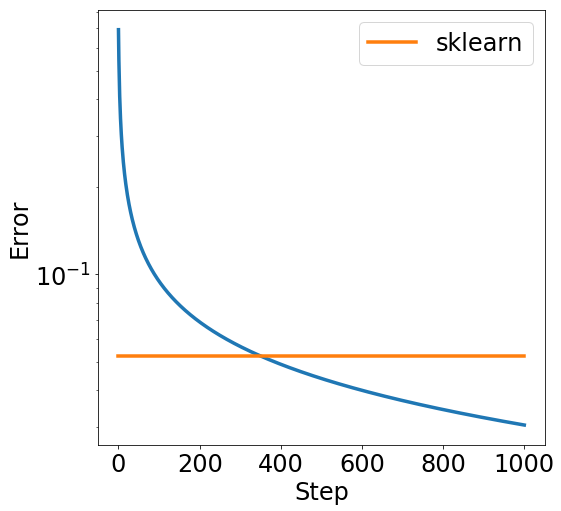In :
error_plot([log_likelihood(w, data, labels) for w in ws_newton])
plt.plot(range(len(ws_newton)), [baseline]*len(ws_newton),
label='sklearn', **kwargs)
plt.legend();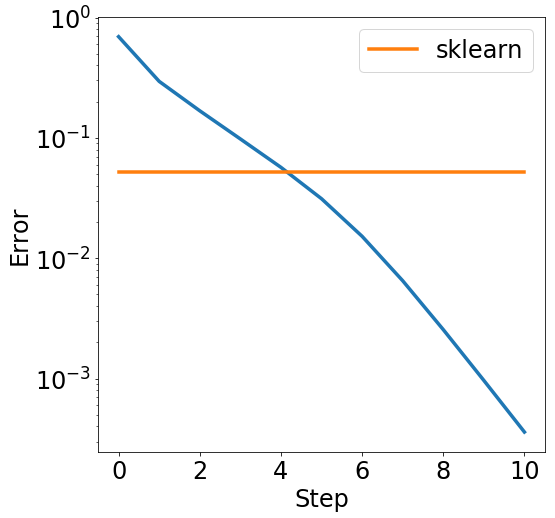## Speed-up via linear equations¶

We'll now see how to speed up Newton's iteration with a simple trick. Instead of computing a full pseudo-inverse, we'll solve set of a linear equations approximately. Recall that the system $Hx=g$ has the solution $x=H^{-1}g$.

In :
def newton_method_lsq(init, steps, grad, hessian, least_squares,
num_to_keep=None):
"""Newton iteration with least squares solver.

Parameters
----------
initial : array
starting point
steps : list of floats
step size schedule for the algorithm
mapping arrays to arrays of same shape
hessian : function
mapping 1d arrays to 2d arrays
least_squares : function
solver for linear equations
num_to_keep : integer, optional
number of points to keep

Returns
-------
List of points computed by Newton iteration. Length of the
list is determined by num_to_keep.
"""
xs = [init]
for step in steps:
# Use least squares solver for Hessian-gradient product
xs.append(xs[-1] - step * direction)
if num_to_keep:
xs = xs[-num_to_keep:]
return xs


If we use a full least squares solve, this is just equivalent to the pseudo-inverse we had before. This why Newton's method for logistic regression is sometimes called iteratively reweighted least squares.

In :
lsq = lambda A, b: np.linalg.lstsq(A, b)
ws_newton_lsq = newton_method_lsq(init, np.ones(10), gradient, hessian, lsq)
error_plot([log_likelihood(w, data, labels) for w in ws_newton_lsq])
plt.plot(range(len(ws_newton_lsq)), [baseline]*len(ws_newton_lsq),
label='sklearn', **kwargs)
_ = plt.legend()Let's plug in an approximate solver to see what happens. All the tools for linear equations we've seen in previous lectures (gradient descent, conjugate gradient etc.) could be used here. We'll just stick to gradient descent to keep it simple.

In :
def lsq_gd(A, b):
"""Approximate least squares with gradient descent"""

ws_newton_lsq_gd = newton_method_lsq(init, np.ones(500), gradient, hessian, lsq_gd)
error_plot([log_likelihood(w, data, labels) for w in ws_newton_lsq_gd])
plt.plot(range(len(ws_newton_lsq_gd)), [baseline]*len(ws_newton_lsq_gd),
label='sklearn', **kwargs)
_ = plt.legend()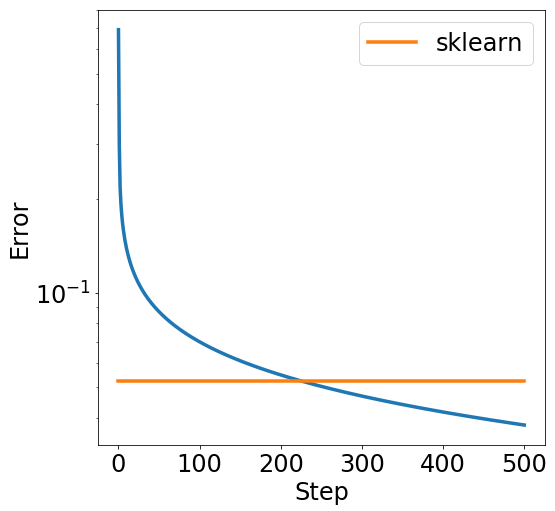It looks like we didn't gain much compared with plain gradient descent. The number of iterations to reach the baseline got smaller, but each iteration is now costlier. Nonetheless, this idea gives rise to set of interesting heuristics.

## BFGS¶

In :
def bfgs(init, grad, hessian, line_search, num_steps, num_to_keep=None):
"""BFGS algorithm (toy implementation).

Parameters
----------
initial : array
starting point
mapping arrays to arrays of same shape
hessian : function
mapping 1d arrays to 2d arrays
line_search : function
method to determine step size
num_steps : number
number of iterations
num_to_keep : integer, optional
number of points to keep

Returns
-------
List of points computed by Newton iteration. Length of the
list is determined by num_to_keep.
"""
xs = [init]
I = np.eye(len(init))
Hs = [hessian(init)]
Hinvs = [I]

for _ in range(num_steps):
# update point
step = line_search(xs[-1], direction)
xs.append(xs[-1] + step * direction)

s = xs[-1] - xs[-2]
ys = np.dot(y, s)
ssT = np.outer(s, s)

# udpate H
H = Hs[-1] + np.outer(y, y)/ys
H -= Hs[-1].dot(ssT.dot(Hs[-1]))/np.dot(s, Hs[-1].dot(s))
Hs.append(H)

# update Hinv
left = I - np.outer(s, y)/ys
right = I - np.outer(y, s)/ys
Hinvs.append(left.dot(Hinvs[-1]).dot(right) + ssT/ys)

if num_to_keep:
xs = xs[-num_to_keep:]

return xs


Let's first try this out with a constant step size.

In :
def line_search(x, d):
"""Constant step size."""
return -0.1

ws_bfgs = bfgs(init, gradient, hessian, line_search, 100)
error_plot([log_likelihood(w, data, labels) for w in ws_bfgs])
plt.plot(range(len(ws_bfgs)), [baseline]*len(ws_bfgs),
label='sklearn', **kwargs)
_ = plt.legend()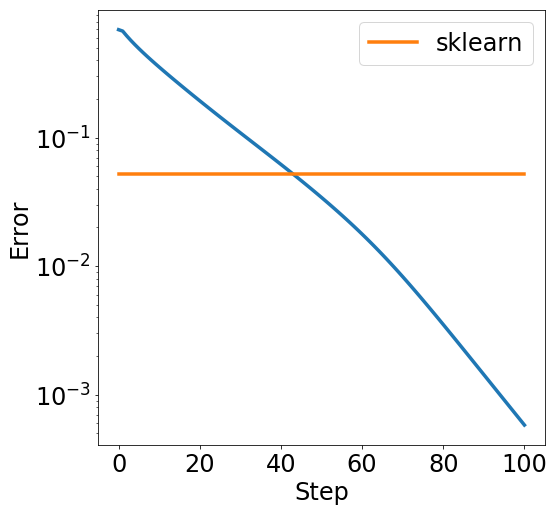Now find a clever step size using the golden section search algorithm we discussed in a previous lecture.

In :
def line_search(x, d):
return gss(lambda step: log_likelihood(x+step*d, data, labels), -1, 1)

ws_bfgs = bfgs(init, gradient, hessian, line_search, 10)
error_plot([log_likelihood(w, data, labels) for w in ws_bfgs])
plt.plot(range(len(ws_bfgs)), [baseline]*len(ws_bfgs),
label='sklearn', **kwargs)
_ = plt.legend()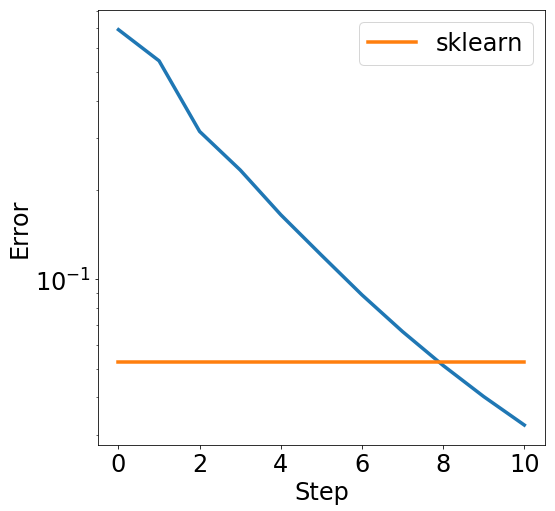We see that BFGS with line search gets pretty close to Newton's method in iteration count, but each iteration is now much cheaper (in terms of the data dimension).

## Hessian-vector products¶

Recall that we can implement the Hessian inverse (approximately or exactly) by just using Hessian vector products. This follows from the Krylov methods for solving linear equations.

This raises the question how we can, in general, compute Hessian-vector products of possibly complicated functions for which we have no exact formula for the Hessian.

Luckily, this problem is also solved by automatic differentiation. Let's import autograd first and define some function. For simplicity we'll reuse the log-likelihood we saw before.

In :
import autograd.numpy as np

np.random.seed(1337)

In :
def log_likelihood(weights, data, labels):
"""Normalized negative log likelihood."""
scores = np.dot(data, weights)
return -np.mean(labels*scores - np.log(1 + np.exp(scores)))

In :
m, n = 1000, 100
data = np.random.normal(0, 1, (m, n))
wopt = np.random.normal(0, 1, n)
labels = (data.dot(wopt) > 0).astype(np.float)


Here's now how to get Hessian vector products with just two invocations of automatic differentiation.

In :
objective = lambda w: log_likelihood(w, data, labels)

In :
vector = np.random.normal(0, 1, n)


Let's verify that hvprod correctly implements the product of the Hessian at a given point with vector.

In :
def log_likelihood_hessian(weights, data, labels):
"""Hessian of negative log likelihood."""
predictions = logistic_model(weights, data)
diag = np.diag(predictions * (1 - predictions))
return data.T.dot(diag.dot(data))/len(data)

In :
H = log_likelihood_hessian(wopt, data, labels)
np.allclose(hvprod(wopt), H.dot(vector))

Out:
True

Let's see how this all plays out on a more realistic data set, the UCI adult data set. We'll first preprocess the data in a standard way.

In :
import pandas as pd
import sklearn as skl
import sklearn.preprocessing as preprocessing
import sklearn.linear_model as linear_model
import sklearn.metrics as metrics
import sklearn.tree as tree

In :
features = ["Age", "Workclass", "fnlwgt", "Education", "Education-Num", "Martial Status",
"Occupation", "Relationship", "Race", "Sex", "Capital Gain", "Capital Loss",
"Hours per week", "Country", "Target"]

num_train = len(original_train)
original = pd.concat([original_train, original_test])
labels = original['Target']
labels = labels.replace('<=50K', 0).replace('>50K', 1)
labels = labels.replace('<=50K.', 0).replace('>50K.', 1)

# Redundant column
del original["Education"]
del original["Target"]

def data_transform(df):
binary_data = pd.get_dummies(df)
feature_cols = binary_data[binary_data.columns[:-2]]
scaler = preprocessing.StandardScaler()
data = pd.DataFrame(scaler.fit_transform(feature_cols), columns=feature_cols.columns)
return data

data = data_transform(original)
train_data = data[:num_train]
train_labels = labels[:num_train]
test_data = data[num_train:]
test_labels = labels[num_train:]

In :
len(train_data), len(test_data)

Out:
(32561, 16281)
In :
original

Out:
Age Workclass fnlwgt Education-Num Martial Status Occupation Relationship Race Sex Capital Gain Capital Loss Hours per week Country
0 39 State-gov 77516 13 Never-married Adm-clerical Not-in-family White Male 2174 0 40 United-States
1 50 Self-emp-not-inc 83311 13 Married-civ-spouse Exec-managerial Husband White Male 0 0 13 United-States
2 38 Private 215646 9 Divorced Handlers-cleaners Not-in-family White Male 0 0 40 United-States
3 53 Private 234721 7 Married-civ-spouse Handlers-cleaners Husband Black Male 0 0 40 United-States
4 28 Private 338409 13 Married-civ-spouse Prof-specialty Wife Black Female 0 0 40 Cuba
5 37 Private 284582 14 Married-civ-spouse Exec-managerial Wife White Female 0 0 40 United-States
6 49 Private 160187 5 Married-spouse-absent Other-service Not-in-family Black Female 0 0 16 Jamaica
7 52 Self-emp-not-inc 209642 9 Married-civ-spouse Exec-managerial Husband White Male 0 0 45 United-States
8 31 Private 45781 14 Never-married Prof-specialty Not-in-family White Female 14084 0 50 United-States
9 42 Private 159449 13 Married-civ-spouse Exec-managerial Husband White Male 5178 0 40 United-States
10 37 Private 280464 10 Married-civ-spouse Exec-managerial Husband Black Male 0 0 80 United-States
11 30 State-gov 141297 13 Married-civ-spouse Prof-specialty Husband Asian-Pac-Islander Male 0 0 40 India
12 23 Private 122272 13 Never-married Adm-clerical Own-child White Female 0 0 30 United-States
13 32 Private 205019 12 Never-married Sales Not-in-family Black Male 0 0 50 United-States
14 40 Private 121772 11 Married-civ-spouse Craft-repair Husband Asian-Pac-Islander Male 0 0 40 NaN
15 34 Private 245487 4 Married-civ-spouse Transport-moving Husband Amer-Indian-Eskimo Male 0 0 45 Mexico
16 25 Self-emp-not-inc 176756 9 Never-married Farming-fishing Own-child White Male 0 0 35 United-States
17 32 Private 186824 9 Never-married Machine-op-inspct Unmarried White Male 0 0 40 United-States
18 38 Private 28887 7 Married-civ-spouse Sales Husband White Male 0 0 50 United-States
19 43 Self-emp-not-inc 292175 14 Divorced Exec-managerial Unmarried White Female 0 0 45 United-States
20 40 Private 193524 16 Married-civ-spouse Prof-specialty Husband White Male 0 0 60 United-States
21 54 Private 302146 9 Separated Other-service Unmarried Black Female 0 0 20 United-States
22 35 Federal-gov 76845 5 Married-civ-spouse Farming-fishing Husband Black Male 0 0 40 United-States
23 43 Private 117037 7 Married-civ-spouse Transport-moving Husband White Male 0 2042 40 United-States
24 59 Private 109015 9 Divorced Tech-support Unmarried White Female 0 0 40 United-States
25 56 Local-gov 216851 13 Married-civ-spouse Tech-support Husband White Male 0 0 40 United-States
26 19 Private 168294 9 Never-married Craft-repair Own-child White Male 0 0 40 United-States
27 54 NaN 180211 10 Married-civ-spouse NaN Husband Asian-Pac-Islander Male 0 0 60 South
28 39 Private 367260 9 Divorced Exec-managerial Not-in-family White Male 0 0 80 United-States
29 49 Private 193366 9 Married-civ-spouse Craft-repair Husband White Male 0 0 40 United-States
... ... ... ... ... ... ... ... ... ... ... ... ... ...
16251 81 NaN 26711 11 Married-civ-spouse NaN Husband White Male 2936 0 20 United-States
16252 60 Private 117909 11 Married-civ-spouse Prof-specialty Husband White Male 7688 0 40 United-States
16253 39 Private 229647 13 Never-married Tech-support Not-in-family White Female 0 1669 40 United-States
16254 38 Private 149347 14 Married-civ-spouse Prof-specialty Husband White Male 0 0 50 United-States
16255 43 Local-gov 23157 14 Married-civ-spouse Exec-managerial Husband White Male 0 1902 50 United-States
16256 23 Private 93977 9 Never-married Machine-op-inspct Own-child White Male 0 0 40 United-States
16257 73 Self-emp-inc 159691 10 Divorced Exec-managerial Not-in-family White Female 0 0 40 United-States
16258 35 Private 176967 10 Married-civ-spouse Protective-serv Husband White Male 0 0 40 United-States
16259 66 Private 344436 9 Widowed Sales Other-relative White Female 0 0 8 United-States
16260 27 Private 430340 10 Never-married Sales Not-in-family White Female 0 0 45 United-States
16261 40 Private 202168 15 Married-civ-spouse Prof-specialty Husband White Male 15024 0 55 United-States
16262 51 Private 82720 9 Married-civ-spouse Craft-repair Husband White Male 0 0 40 United-States
16263 22 Private 269623 10 Never-married Craft-repair Own-child White Male 0 0 40 United-States
16264 64 Self-emp-not-inc 136405 9 Widowed Farming-fishing Not-in-family White Male 0 0 32 United-States
16265 50 Local-gov 139347 14 Married-civ-spouse Prof-specialty Wife White Female 0 0 40 NaN
16266 55 Private 224655 9 Separated Priv-house-serv Not-in-family White Female 0 0 32 United-States
16267 38 Private 247547 11 Never-married Adm-clerical Unmarried Black Female 0 0 40 United-States
16268 58 Private 292710 12 Divorced Prof-specialty Not-in-family White Male 0 0 36 United-States
16269 32 Private 173449 9 Married-civ-spouse Handlers-cleaners Husband White Male 0 0 40 United-States
16270 48 Private 285570 9 Married-civ-spouse Adm-clerical Husband White Male 0 0 40 United-States
16271 61 Private 89686 9 Married-civ-spouse Sales Husband White Male 0 0 48 United-States
16272 31 Private 440129 9 Married-civ-spouse Craft-repair Husband White Male 0 0 40 United-States
16273 25 Private 350977 9 Never-married Other-service Own-child White Female 0 0 40 United-States
16274 48 Local-gov 349230 14 Divorced Other-service Not-in-family White Male 0 0 40 United-States
16275 33 Private 245211 13 Never-married Prof-specialty Own-child White Male 0 0 40 United-States
16276 39 Private 215419 13 Divorced Prof-specialty Not-in-family White Female 0 0 36 United-States
16277 64 NaN 321403 9 Widowed NaN Other-relative Black Male 0 0 40 United-States
16278 38 Private 374983 13 Married-civ-spouse Prof-specialty Husband White Male 0 0 50 United-States
16279 44 Private 83891 13 Divorced Adm-clerical Own-child Asian-Pac-Islander Male 5455 0 40 United-States
16280 35 Self-emp-inc 182148 13 Married-civ-spouse Exec-managerial Husband White Male 0 0 60 United-States

48842 rows × 13 columns

In :
X = train_data.values
y = train_labels.values

In :
objective = lambda w: likelihood(w, X, y)
hessian = lambda w: log_likelihood_hessian(w, X, y)
init = np.zeros(X.shape)

cls = linear_model.LogisticRegression()
cls.fit(X, y)
weights = np.reshape(cls.coef_, cls.coef_.shape)
baseline = log_likelihood(weights, X, y)

ws_newton = newton_method(init, np.ones(10), gradient, hessian)
error_plot([log_likelihood(w, X, y) for w in ws_newton])
plt.plot(range(len(ws_newton)), [baseline]*len(ws_newton),
label='sklearn', **kwargs)
_ = plt.legend()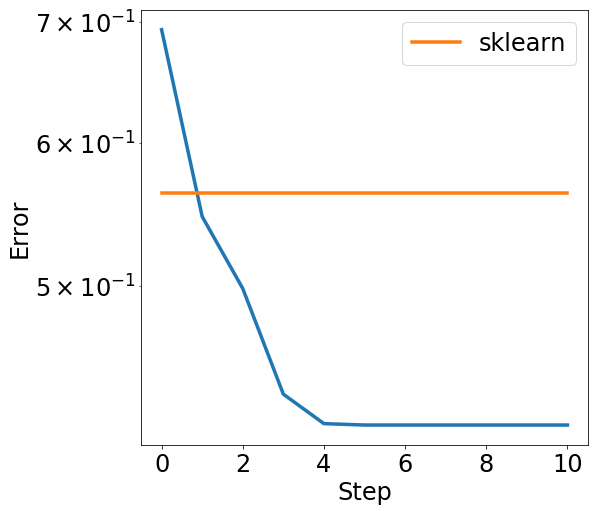In :
def line_search(x, d):
return gss(lambda step: log_likelihood(x+step*d, X, y), -1, 1, tol=0.1)

ws_bfgs = bfgs(init, gradient, hessian, line_search, 10)
error_plot([log_likelihood(w, X, y) for w in ws_bfgs])
plt.plot(range(len(ws_bfgs)), [baseline]*len(ws_bfgs),
label='sklearn', **kwargs)
_ = plt.legend()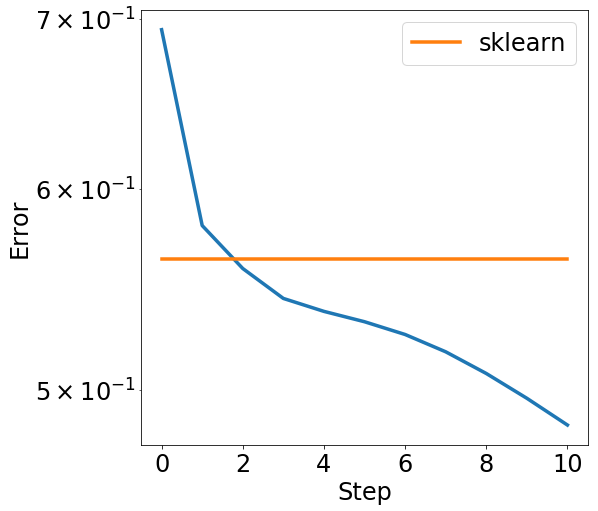### Timing comparison¶

In :
timeit(lambda: linear_model.LogisticRegression().fit(X, y), number=1)

Out:
1.6104111671447754
In :
timeit(lambda: bfgs(init, gradient, hessian, line_search, 10), number=1)

Out:
6.190078973770142
In :
timeit(lambda: gradient_descent(init, np.ones(200), gradient), number=1)

Out:
1.7400920391082764

### Accuracy on the test set¶

In :
Xtest = test_data.values
ytest = test_labels.values

In :
def accuracy(weights):
predictions = logistic_model(weights, Xtest)
return np.mean((predictions > 0.5).astype(np.float) == ytest)

In :
ws_gd = gradient_descent(init, np.ones(500), gradient)
accuracy(ws_gd[-1])

Out:
0.82636201707511825
In :
ws_bfgs = bfgs(init, gradient, hessian, line_search, 20)
accuracy(ws_bfgs[-1])

Out:
0.83139856274184631
In :
cls = linear_model.LogisticRegression(C=1, fit_intercept=False)
cls.fit(X, y)
cls.score(Xtest, ytest)
weights = np.reshape(cls.coef_, cls.coef_.shape)
accuracy(weights)

Out:
0.8308457711442786

We see that our toy implementation of BFGS is actually fairly competitive. So is gradient descent which reaches good test performance with an optimized constant step size.